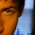### Order of operations / PEMDASPEMDAS does not cover matrices... Photo courtesy of Stuartpilbrow

Could you please share with me your opinion of the "Please Excuse My Dear Aunt Sally" simplifying expressions. Any feedback could you give will be appreciated. Thank you.

This "PEMDAS" rule is a mnemonic for order of operations:

Excuse = Exponents
My = Multiplication
Dear = Division
Sally = Subtraction

There's nothing wrong with using a mnemonic to remember the order of operations. However, one has to bear in mind that

1. This rule is not all-inclusive. It omits for example square roots. But the rule is good for all elementary grades. (Square roots would be on the same level or rank with exponents, by the way.)

2. The rule doesn't spell out the fact that in reality multiplication and division are "on the same level" or rank. This means that if you have several multiplications and divisions, you do them from left to right, and not "multiplication first, then division".

For example: 60 ÷ 5 × 4. You go from left to right, and first do 60 ÷ 5 = 12. Then you multiply 12 × 4 = 48.

If you want 5 × 4 to be done first, it needs to be in parenthesis: 60 ÷ (5 × 4). Here, first do 5 × 4 = 20, and then 60 ÷ 20 = 3.

Similarly, addition and subtraction are on the same level: if both exist in an expression, they are to be done from left to right.

An example: Simplify the expression 2 × 5 − 6 + 8.

1: Multiply 2 × 5 = 10.
The expression is now 10 − 6 + 8.

2. Subtract. 10 − 6 = 4.
The expression is now 4 + 8.

3. Add. 4 + 8 = 12.

So, perhaps it's more illustrative to lay out the PEMDAS rule like this:

Excuse = Exponents
My Dear = Multiplication & Division
Aunt Sally = Addition & Subtraction

...and say it with little pauses at the commas: Please, Excuse, My Dear, Aunt Sally.

3. Fraction line as a division symbol generates implicit groupings or parenthesis in the fact that anything written on top or bottom of the fraction line is to be done before the division. It's as if the whole numerator and denominator were inside parenthesis.

So...

```4 × 32
--------
7 − 22
```

means the same as (4 × 32) ÷ (7 − 22). Simplifying this is left as an exercise for the reader. The answer is 12.

See also a detailed lesson on order of operations at Purplemath and Wikipedia's note on mnemonics.Sundayteatime said…
In the UK we use BIDMAS - Brackets, Indices, Division, Multiplication, Addition, Subtraction. Seems to work quite well.Frnk Ho said…
The fractions situation will follow the reduciing rule that is if top and bottom are all in product of factors then they can reduced by dividing common factors. So it is a bit different from the linear fashion of Order of Operations.Mathchart said…
If you leave out the rule that multiplication and division are at the same level and are then performed in left to right order, the student thinks that multiplication must be performed before division i.e. peMDas. PEMDAS is helpful, but you must mention the left to right rule for operations of the same level.pam said…
In teaching algebra, I made use of the mnemonic PEMDAS by switching it to SADMEP when solving. This helped the students connect their prior knowledge of order of operations with the skills needed to "get the variable by itself". We started by peeling off any addition or subtraction, then multiplication or division, etc. Doing PEMDAS backwards as SADMEP also helped connect the idea of doing the opposite of each operation to move it to the other side.I use in my school a diagram. i draw in the board a ladder and then start from the top like this:
parenthesis (top step)
exponents and square roots (next step going down)
multiplication and division (next step)
equal sign (floor)

excuse my English but it is not my native language.Unknown said…
I ask my students to write their own mnemonic to remember the order of operations. One of the nice ones was Purple Eggplants Make Delicious Afternoon Snack. I encourage my students to say: Multiplication and Division whichever comes first from left to right. Addition and subtraction whichever comes first from left to right.Anonymous said…
dear Maria
I liked your mnemonic PEMDAS a lot I think students will find it fun to work withI think the use of a mnemonic device is useful, but with a caveat when teaching the order of operations. I teach 7th grade and unfortunately, while the students know the phrase "Please excuse my dear Aunt Sally" -- there seems to NOT have been enough stress placed on the rule that students must multiply or divide AS IT OCCURS left to right, or add or substract AS IT OCCURS left to right.Unknown said…
Have you ever wondered why we were excusing dear Aunt Sally?

Excuse
My Dear
Aunt Sally

For Looking Too Rough
(From Left To Right)

when I teach order of operations I use this to remind students to do the Multiplication & Division and the Addition & Subtraction from left to right.
They get a kick out of knowing why we’re excusing Aunt Sally.Drew said…
Funny video on the Order of Operations: http://www.youtube.com/watch?v=GhQ-Yzj0buk